American Journal of Modern Energy
Volume 1, Issue 1, June 2015, Pages: 1-16

Heat Generation/Absorption Effect on Natural Convection Heat Transfer in a Square Enclosure Filled with a Ethylene Glycol - Copper Nanofluid Under Magnetic Field

Mohamed Bechir Ben Hamida1, Kamel Charrada2

1Laboratory of Ionized Backgrounds and Reagents Studies (LEMIR), High School of Sciences and Technology of Hammam Sousse (ESSTHS), University of Sousse, Sousse, Tunisia

2Laboratory of Ionized Backgrounds and Reagents Studies (LEMIR), Preparatory Institute for Engineering Studies of Monastir (IPEIM), University of Monastir, Monastir, Tunisia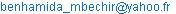(M. B. B. Hamida)

Mohamed Bechir Ben Hamida, Kamel Charrada. Heat Generation/Absorption Effect on Natural Convection Heat Transfer in a Square Enclosure Filled with a Ethylene Glycol - Copper Nanofluid Under Magnetic Field. American Journal of Modern Energy. Vol. 1, No. 1, 2015, pp. 1-16. doi: 10.11648/j.ajme.20150101.11

Abstract: This paper examines the natural convection in a square enclosure that is filled with a nanofluid. This nanofluid with Ethylene Glycol based containing Copper nanoparticle is influenced by a uniform horizontal magnetic field and uniform heat generation or heat absorption. The enclosure is bounded by two isothermal vertical walls at different temperatures and by two horizontal adiabatic walls. The governing equations needed to deal this problem (mass, momentum, and energy) are solved numerically using the commercial simulation software COMSOL Multiphysics. In order to increase the natural convective heat transfer in a square cavity, the effect of heat generation or absorption on the isothermal, streamline contours and the Nusselt number are studied when the Prandtl number is Pr = 151.

Keywords: Generation/Absorption, Comsol Multiphysics

1. Introduction

The study of magnetohydrodynamic flow when especially associated with heat transfer has attracted several researchers in recent years due to its wide variety of applications in engineering areas and technology such as plasma industries, cooling of nuclear reactor, optimization of crystal growth processes, electronic package, geothermal energy extractions, solidification of metal alloys, metallurgical applications involving casting and solar technology.

The existence of an external magnetic field is used as a control mechanism in material manufacturing industry, as the convection currents are suppressed by Lorentz force which is produced by the magnetic field.

Rudraiah et al.  studied numerically the natural convection of an electrically conducting fluid in the presence of a magnetic field parallel to gravity. They have pointed out that the average Nusselt number decreases with an increase in the Hartmann, and the Nusselt number approaches one under a strong magnetic field. Piazza and Ciofalo  considered the buoyancy-driven magnetohydrodynamic flow in a liquid-metal-filled cubic enclosure differentially heated at two opposing vertical walls. Kandaswamy et al.  studied the magnetoconvection flow in a enclosure with partially active vertical walls. They have considered nine different positions of active zone for different value of the Rayleigh and Hartman numbers. It has been found that the average Nusselt number decreases with an increase of Hartman number and increases with the Rayleigh number. Also, the convection mode of heat transfer is converted into a conduction mode for an enough large magnetic field. Ghassemi et al.  investigated the effect of the magnetic field on natural convection in a enclosure with two adiabatic baffles. They showed that the magnetic field had an adverse effect on the Nusselt number, particularly at low values of Rayleigh number. Pirmohammadi and Ghassemi  considered the effect of the magnetic field on convection heat transfer inside a tilted square enclosure. Their study showed that the heat transfer mechanism and flow characteristics inside the enclosure depend strongly upon both magnetic field and inclination angle. Sathiyamoorthy and Chamkha  investigated natural magneto-convection of liquid Gallium in a square heated cavity. They found that the average Nusselt number decreased nonlinearly with the Hartman number. Sivasankaran and Ho  studied numerically the effects of temperature dependent properties on the natural convection of water in a enclosure under the influence of a magnetic field. They showed that the heat transfer rate decreases with an increase of the magnetic field and influenced by the direction of the external magnetic field. Sarris et al  investigated the natural convection of an electrically conducting fluid in a laterally and volumetrically heated square cavity under the influence of a magnetic field. They concluded that the heat transfer is enhanced with increasing internal heat generation parameter, but no significant effect of the magnetic field is observed due to the small range of the Hartmann numbers. Bhuvaneswari et al.  investigated magnetic convection in an enclosure with non--uniform heating on both walls. They found that the heat transfer rate is increased on increasing the amplitude ratio. Kolsi et al  studied the effect of magnetic field orthogonal to the isothermal walls on the unsteady natural convection in a differentially heated cubic cavity. They observed the role of magnetic field on the damping and laminarization of flow field.

The main fluids used for heat transfer application are water, ethylene glycol, mineral oil or propylene glycol have a low thermal conductivity. In order to improve the heat transfer performance of inherently poor conventional heat transfer base fluids, the use of nanofluids is one of the most effective mechanisms which has a superior thermal conductivity compared to the base fluid.

Many publications, either theoretical [11,12] or experimental [13-16], use the water as base fluid with several particles such as Al2O3 [17,18], Cu  , CuO , TiO2 and Ag. But, a few research work on ethylene glycol [21-26] as base fluid despite its extensive application in industrial and energy saving perspectives such as in power generation, heating and cooling processes, heat exchanger, chemical processes, electronics, transportation, automotive and others.

In addition, the influence of a heat generation in a cavity containing only base fluid without nanofluids was studied by a few researchers such as Khanafer et al.  and Hossain et al. .

Therefore, The main originality of the present study is to investigate in detail the natural convection in a square enclosure by utilizing Ethylene Glycol - Copper Nanofluid and is influenced by a horizontally applied uniform magnetic field in the presence of uniform heat generation or absorption. The physical models are solved using the commercial simulation software COMSOL Multiphysics. The results of the simulation are presented and discussed in the sections below.

2. Numerical Model

2.1. Simplifying Assumptions

The governing equations of the numerical model are written taking into account the following simplifying assumptions:

The base fluid Ethylene Glycol and the nanoparticles are assumed in thermal equilibrium.

The nanofluid is Newtonian and incompressible.

The flow is considered to be steady, two-dimensional and laminar.

The displacement currents, induced magnetic field, dissipation and joule heating are neglected.

2.2. Governing Equations

2.2.1. Equations of the Model in Dimensional Form

The governing equations for this problem are based on the balance laws of mass, momentum and thermal energy of a steady laminar flow. Taking into account the assumptions previously-mentioned, the simplified system of equations is written in two-dimensional form as follows:

Mass conservation equation: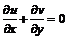(1)

Where u andv are the velocities components in the x and the y direction, respectively.

Momentum conservation equation in the x direction :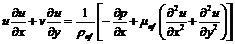(2)

Where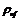, p andare the density of nanofluid, fluid pressure and the effective dynamic of the nanofluid, respectively.

Momentum conservation equation in the y direction :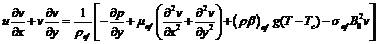(3)

Where Tc is the temperature of cold wall ,is the thermal expansion coefficient,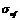is the electrical conductivity of nanofluid and B0 is the magnetic field strength.

Energy conservation equation: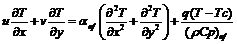(4)

Where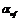is the thermal diffusivity of nanofluid and q is the heat generation or the heat absorption.

The properties of the nanofluid used in this study are inspired by classical models reported in the literature .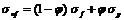(5)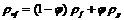(6)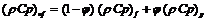(7)(8)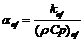(9)

In the above equations, k is the thermal conductivity, Cp is the specific heat and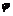is the solid volume fraction. The subscripts f and p means the fluid and nanoparticle, respectively.

The thermal conductivity and the effective dynamic viscosity of the nanofluid can be modelled by [30,31].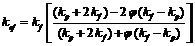(10)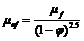(11)

2.2.2. Equations of the Model in Non-Dimensional Form

The governing equations are nondimensionalized using the following variables: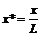,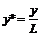,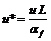,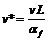,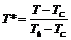,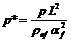,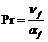,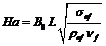,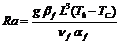and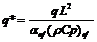Based on the presumptions above, the dimensionless governing equations can be expressed as follows:

Mass conservation equation: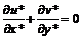(12)

Momentum conservation equation in the x direction :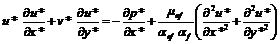(13)

Momentum conservation equation in the y direction :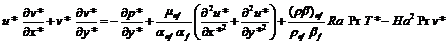(14)

Energy conservation equation: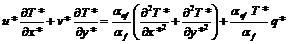(15)

These previous equations are solved using the commercial simulation software COMSOL MULTIPHYSICS.

2.2.3. Nusselt Number Calculations

The local Nusselt number on the left hot wall can be defined as :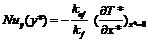(16)

The average Nusselt number (Num) is calculated by integrating Nuy along the hot wall :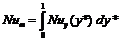(17)

The stream function is determinated from :et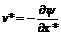(18)

2.3. Boundary Conditions of the Model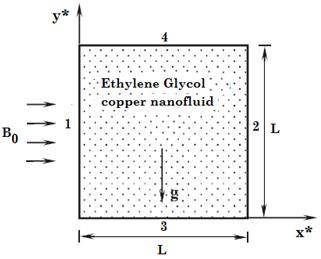Figure 1. Schematic diagram of the physical model.

The schematic of a two-dimensional square cavity is shown in Figure 1. It is filled with an electrically conducting fluid and a Ethylene Glycol - Copper nanofluid. The left and right side walls are kept at constant temperatures, TC and Th, respectively. The top and bottom surfaces are assumed to be insulated and impermeable. A magnetic field with uniform strength B0 is applied in the x direction which is perpendicular to the gravity.

The boundary conditions used for dimensional and dimensionless form are summarized to the Table 1.

Table 1. Boundary conditions for dimensional and dimensionless form.

 Border Dimensional form Dimensionless form Condition on u Condition on v Condition on T Condition on u* Condition on v* Condition on T* 1 0 0 Th 0 0 1 2 0 0 TC 0 0 0 3 0 0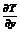0 0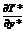4 0 0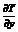0 0The thermo-physical properties of base fluid (Ethylene Glycol: C2H6O2) [32,25] with Copper  (Cu), Alumina  (Al2O3), Titanium oxide (TiO2) and Copper oxide (CuO)  are given in Table 2.

Table 2. Thermo-physical properties of Ethylene Glycol and nanoparticles.

 Pr ρ [kg/m3] Cp [J/kg.K] K [W/m.K]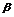×10-5  [K-1]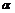×10-5[m2/s] Base fluid Ethylene Glycol (C2H6O2) 151 1109 2400 0.26 65 0.0214 Nano- particles Copper (Cu) 8933 385 401 1.67 11.7 Alumina (Al2O3) 3970 765 40 0.85 1.3 Titanium oxide (TiO2) 4250 686.2 8.9538 0.9 0.31 Copper oxide (CuO) 6500 535.6 20 0.85 0.57

3. Validation of the Model

In order to validate the present numerical method for natural convection in an enclosure, comparisons with the experimental results from Linthorst et al.  are made. They measured the dimensionless y-direction velocity by means of a laser doppler velocimeter at midplane (y* = 0.5) of a enclosure filled with air. For the computational validation, the Rayleigh numbers are set to 1.3 × 105. A square cavity with a length L = 4 cm, whose upper and bottom walls are adiabatics, is chosen. The Prandtl number of the air is fixed as 0.733 while the thermal diffusivity is 26.89 × 106 m2/s, which was deduced from the mean temperature of fluid equal to 320 K. The computational domain is divided by 51 × 51 uniform grids spatially and the same grids are used for further investigation.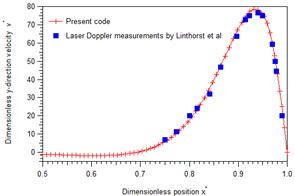Figure 2. Comparison of dimensionless y-direction velocitiesat midplane of a enclosure for Rayleigh number Ra = 1.3 × 105.

The figure 2 shows the dimensionless y-direction velocity according to dimensionless position x* at midplane of a cavity for Raygleigh number Ra = 1.3 × 105 between the theoretical values of our code and the values measured by Linthorst et al. . From this figure, we notice the quite satisfactory agreement between the calculated and measured values, thereby justifying the different assumptions adopted.

4. Results and Discussion

In this study, the Prandtl number is assumed to be Pr = 151. The Rayleigh number (Ra), the Hartmann number (Ha), the dimensionless heat generation or absorption and the solid volume fraction (Ø) are assumed to be in the following ranges: 103 Ra 107, 0 ≤ Ha ≤ 80 , -12 ≤ q* 12 and 0 Ø 0.06. The effect of Hartmann number and Rayleigh number on the isothermal and streamline contours, effect of magnetic field on the Nusselt number, effect of heat generation or absorption and Rayleigh number on the isothermal and streamline contours, effect of heat generation or absorption and Hartmann number on the Nusselt number and the comparison with other nanofluids are presented and discussed.

4.1. Effect of Hartmann Number and Rayleigh Number on the Isothermal and Streamline Contours

Figure 3 shows the isotherms for different Hartmann number (Ha = 0, 20, 40, 60 and 80) and Rayleigh number (Ra = 103, 104, 105, 106 and 107) at dimensionless heat generation q* = 2, a solid volume fraction Ø = 0.05 and Prandlt number Pr = 151.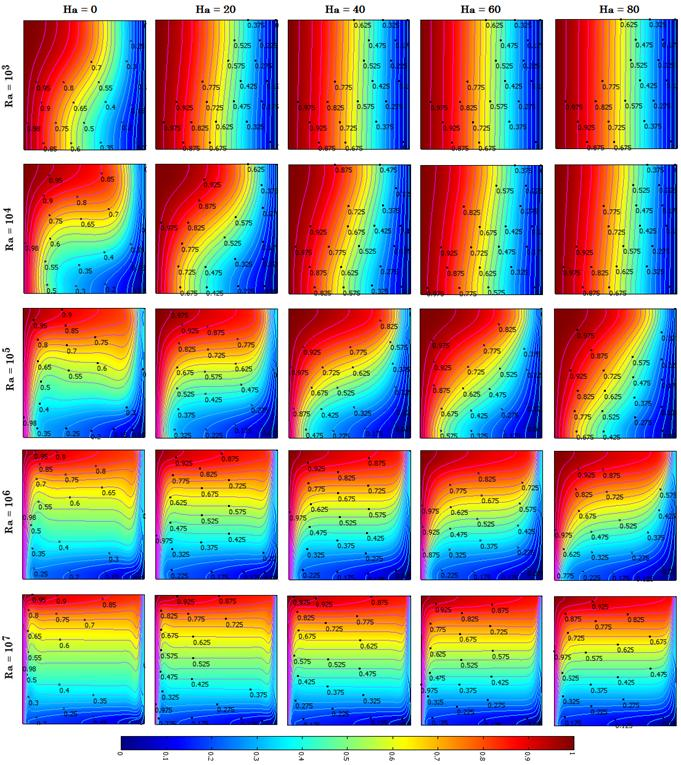Figure 3. Isotherms for different Hartmann number and Rayleigh number at dimensionless heat generation q* = 2 and a solid volume fraction Ø = 0.05 (Ethylene Glycol - Cu nanofluid).

Figure 4 shows the streamlines for different Hartmann number (Ha = 0, 20, 40, 60 and 80) and Rayleigh number (Ra = 103, 104, 105, 106 and 107) at dimensionless heat generation q* = 2, a solid volume fraction Ø = 0.05 and Prandlt number Pr = 151.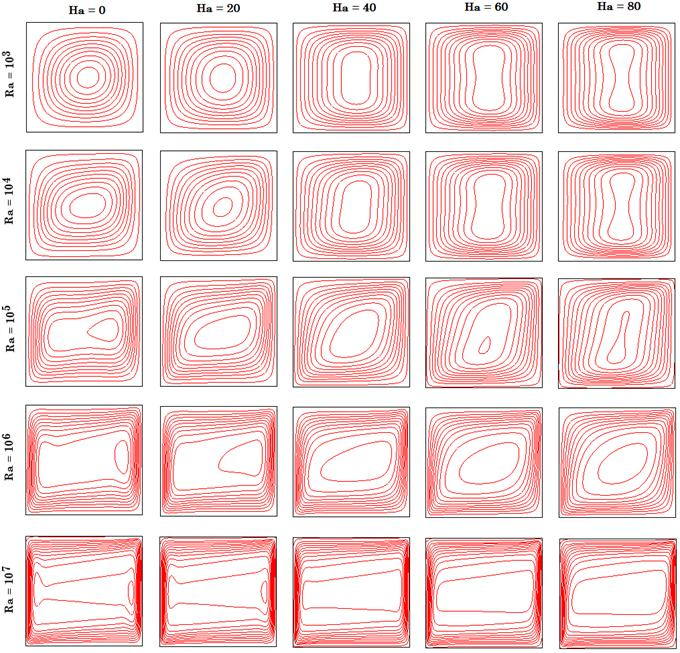Figure 4. Streamlines for different Hartmann number and Rayleigh number at dimensionless heat generation q* = 2 and a solid volume fraction Ø = 0.05 (Ethylene Glycol - Cu nanofluid).

From these figures, we observe that that there is conduction dominated regime at low Rayleigh numbers and a convection dominated regime at high Rayleigh numbers. Indeed, for low Rayleigh number Ra = 103, the isotherms are parallel vertical lines with a weak clockwise circulation in the cavity. Also, we watch that the circulations strength decreases with the increasing of the Hartmann number and increases when the Rayleigh number increases. In addition, the sign of Hartmann number is opposite to the sign of Rayleigh number in source term of equation (14). So, there is an opposite effect of these two parameters on flow regime and Nusselt number. At Rayleigh number Ra = 105, the convection mode is pronounced, the isotherms change from horizontal to vertical when the Hartmann numbers increase. Also, the flow cell becomes stronger. At higher Rayleigh number 107, the convection is dominant and the circulating cell becomes very strong. Also, the streamlines are crowded near the enclosure wall and the core is empty. As well as isothermals are stratified in vertical direction except near the insulated surfaces of the cavity and appear as horizontal lines in the enclosure core.

4.2. Effect of Magnetic Field on the Nusselt Number

Figure 5 shows the Average Nusselt number according to the Raygleigh number (Ra = 103, 104, 105, 106 and 107 ) for different Hartmann number (Ha = 0, 20, 40, 60 and 80) at dimensionless heat generation q* = 2, a solid volume fraction Ø = 0.05 and Prandlt number Pr = 151.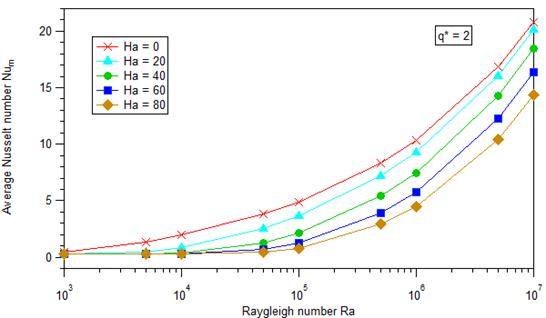Figure 5. Average Nusselt number according to the Raygleigh number for different Hartmann number at dimensionless heat generation q* = 2 and a solid volume fraction Ø = 0.05 (Ethylene Glycol - Cu nanofluid).

Figure 6 shows the ratio between average Nusselt number and average Nusselt number without magnetic field Num/Num(Ha=0) according to the Raygleigh number (Ra = 103, 104, 105, 106 and 107 ) for different Hartmann number (Ha = 0, 20, 40, 60 and 80) at dimensionless heat generation q* = 2, a solid volume fraction Ø = 0.05 and Prandlt number Pr = 151.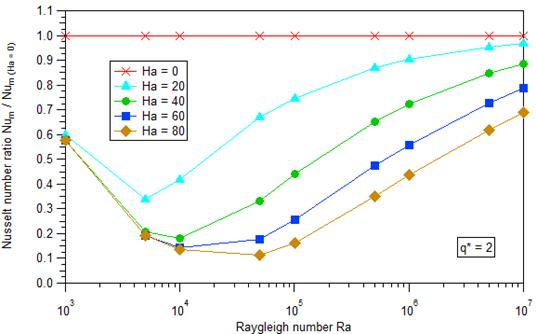Figure 6. Nusselt number ratio according to the Raygleigh number for different Hartmann number at dimensionless heat generation q* = 2 and a solid volume fraction Ø = 0.05 (Ethylene Glycol - Cu nanofluid).

From these figures, we see that for constant Hartmann number, the Nusselt number increases with the increasing of the Raygleigh number. Also, for constant Rayleigh number, the Nusselt number decreases when the Hartmann number increases.

In addition, due to effect of magnetic field, there are two different phases when the Raygleigh number increases. The first, the Nusselt number ratio decreases to minimum value at critical Rayleigh number depending on the Hartmann number. The second, due to the strong effect of the natural convection with respect to the effect of magnetic field, this ratio increases.

4.3. Effect of Heat Generation or Absorption and Rayleigh Number on the Isothermal and Streamline Contours

Figure 7 and 8 show the isotherms for dimensionless heat absorption, dimensionless heat generation and different Rayleigh at Hartmann number Ha = 40, a solid volume fraction Ø = 0.05 and Prandlt number Pr = 151.

Figure 9 and 10 show the streamlines for dimensionless heat absorption, dimensionless heat generation and different Rayleigh at Hartmann number Ha = 40 and a solid volume fraction Ø = 0.05 and Prandlt number Pr = 151.Figure 7. Isotherms for q* ≤ 0 and different Rayleigh at Hartmann number Ha = 40 and a solid volume fraction Ø = 0.05 (Ethylene Glycol - Cu nanofluid).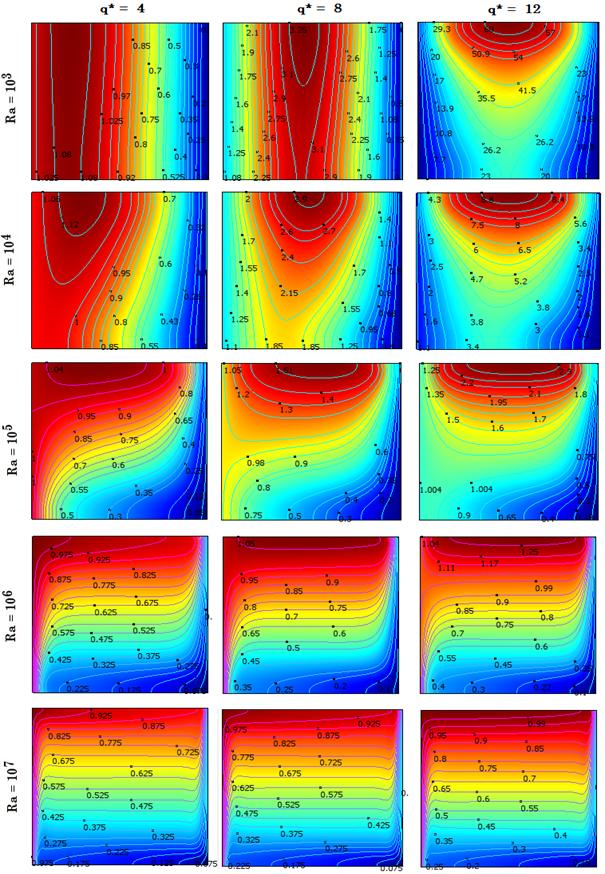Figure 8. Isotherms for q* > 0 and different Rayleigh at Hartmann number Ha = 40 and a solid volume fraction Ø = 0.05 (Ethylene Glycol - Cu nanofluid).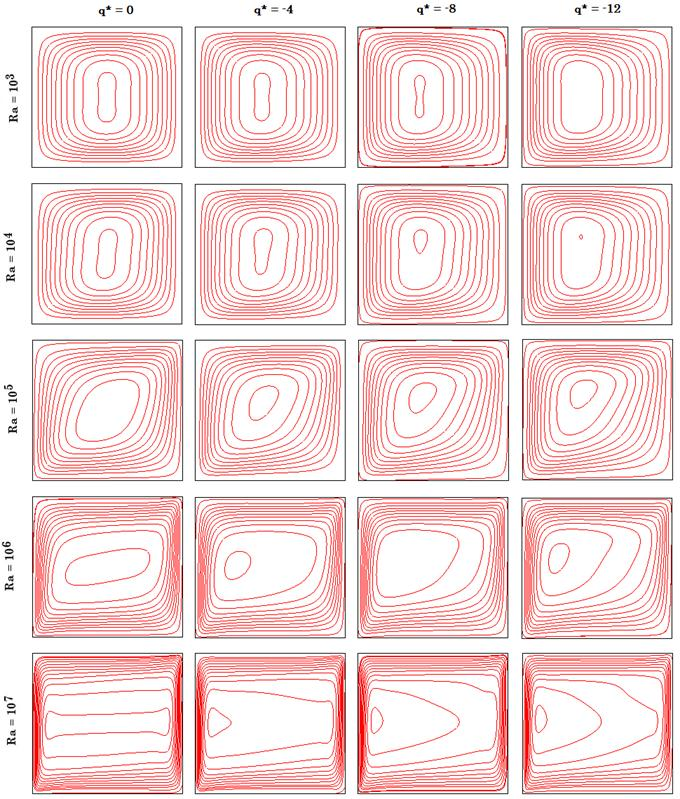Figure 9. Streamlines for q* ≤ 0 and different Rayleigh at Hartmann number Ha = 40 and a solid volume fraction Ø = 0.05 (Ethylene Glycol - Cu nanofluid).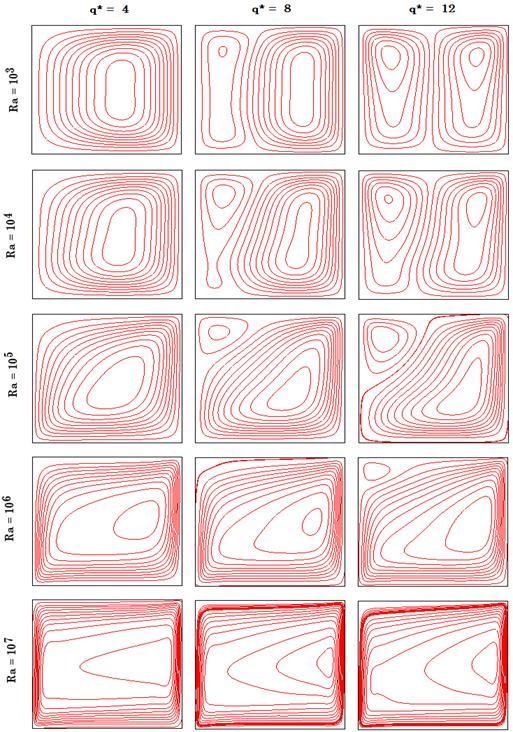Figure 10. Streamlines for q* > 0 and different Rayleigh at Hartmann number Ha = 40 and a solid volume fraction Ø = 0.05 (Ethylene Glycol - Cu nanofluid).

From these figures, we see that the conduction regime is dominant with vertical isotherms for the low Rayleigh number Ra = 103. Also, we observed a strong clockwise circulation in the cavity for a heat sink condition (q* = - 12). But, the strength is reduced gradually when the dimensionless heat increases until high heat generation condition (q* = 12). In the latter case, we notice a higher temperature of nanofluid in the cavity which reduces the rate of heat transfer, Nusselt number and the temperature gradient near the hot wall. Indeed, the two vertical walls temperature is lower than the nanofluids temperature. Therefore, the cold and hot walls receive heat from the nanofluids and a negative Nusselt number at hot wall is found.

In addition, the flow cell becomes stronger when Rayleigh number increases to 105 which the convection mode begins to appear. But, this flow cell is reduced when the dimensionless heat q* increases.

The convection mode begins to appear when Rayleigh number increased to 105. The flow cell becomes stronger but reduces as q* increases.

The convection is become dominant when the Rayleigh number reaches the value of 107. The circulating cell is become very strong with a neglected or small for heat generation.

The temperature gradient near the cavity walls without heat sink condition is lower than that with heat sink. Indeed, we observed, in heat sink condition, that the cavity temperature is lower than the temperature in the cavity without heat sink.

4.4. Effect of Heat Generation or Absorption and Hartmann Number on the Nusselt Number

4.4.1. Effect of Heat Generation or Absorption at Constant Hartmann Number

Figure 11 shows the average Nusselt number according to the Rayleigh number for different dimensionless both heat absorption and heat generation at Hartmann number Ha = 40 and a solid volume fraction of Ø = 0.05 and Prandlt number Pr = 151.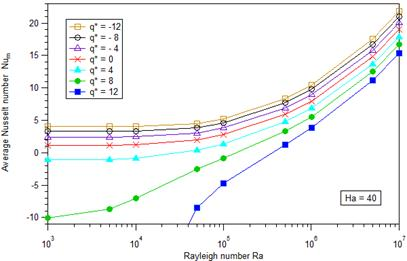Figure 11. Average Nusselt number according to the Rayleigh number for different dimensionless both heat absorption and heat generation at Hartmann number Ha = 40 and a solid volume fraction of Ø = 0.05 (Ethylene Glycol - Cu nanofluid).

From this figure, we see that, for constant Rayleigh number, when the dimensionless heat q* decreases the Nusselt number increases and conversely. Also, for small Rayleigh number, the effect of heat generation (q* > 0) overcomes the effect of natural convection lead to small heat transfer from hot to cold wall or can causes reverse heat transfer from nanofluid to both vertical wall which has a negative Nusselt number. In other word, the effect of both heat generation or absorption is neglected when Rayleigh number increases.

To study well the effect of heat generation or absorption on the Nusselt number, the Nusselt number ratio (the ratio between Nusselt number and Nusselt number without heat generation Num/Num(q*=0) according to the Rayleigh number for different dimensionless both heat absorption and heat generation at Hartmann number Ha = 40 and a solid volume fraction of Ø = 0.05 is shown in Figure 12.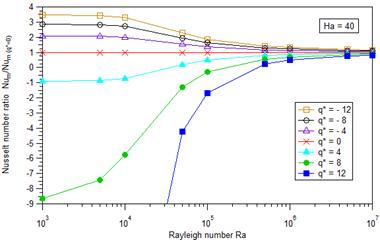Figure 12. Nusselt number ratio according to the Rayleigh number for different dimensionless both heat absorption and heat generation at Hartmann number Ha = 40 and a solid volume fraction of Ø = 0.05 (Ethylene Glycol - Cu nanofluid).

From this figure, we see that, for all Rayleigh number and the heat generation, the Nusselt number ratio is less than one but it is greater than unity for the case of heat absorption. Also, for small Rayleigh Ra = 103, the heat generation or absorption has a major effect on the Nusselt number ratio. This effect decreases with increasing the number of Rayleigh until his neglect at Ra = 107.

4.4.2. Effect of Heat Generation or Absorption at Constant Rayleigh Number

Figure 13 shows the average Nusselt number according to the Hartmann number for different dimensionless both heat absorption and heat generation at a solid volume fraction of Ø = 0.05, Rayleigh number Ra = 105 and Prandlt number Pr = 151.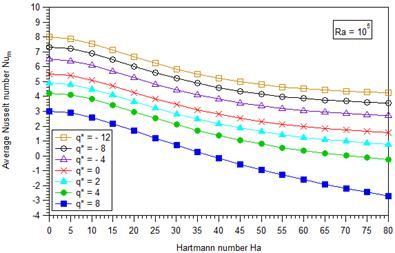Figure 13. Average Nusselt number according to the Hartmann number for different dimensionless both heat absorption and heat generation at Rayleigh number Ra = 105 and solid volume fraction of Ø = 0.05 (Ethylene Glycol - Cu nanofluid).

From this figure, we always see that the Nusselt number for the case of heat source is lower than the case of heat of absorption. Also, for both heat source or heat absorption, the Nusselt number decreases by increasing the number of Hartmann. Therefore, the increasing of the magnetic field reduces the Nusselt number. This reduction is important for the case of heat generation compared to the case of heat absorption. On the other hand, the effect of magnetic field is small in the case of heat absorption but it is greater in the case of heat generation.

Figure 14 shows the Nusselt number ratio according to the Hartmann number for different dimensionless both heat absorption and heat generation at a solid volume fraction of Ø = 0.05, Rayleigh number Ra = 105 and Prandlt number Pr = 151.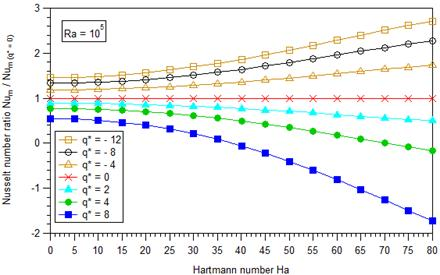Figure 14. Nusselt number ratio according to the Hartmann number for different dimensionless both heat absorption and heat generation at Rayleigh number Ra = 105 and solid volume fraction of Ø = 0.05 (Ethylene Glycol - Cu nanofluid).

From this figure, we see that, for the situation of the heat absorption, the Nusselt number ratio is greater than unity and increases as the Hartmann number increases. Therefore, the increasing of the magnetic field raises the Nusselt number ratio. Then, for the case of the heat generation, the Nusselt number ratio is less than unity and decreases as the Hartmann number increases. Therefore, the increasing of the magnetic field reduces the Nusselt number ratio.

4.5. Comparison with Other Nanofluids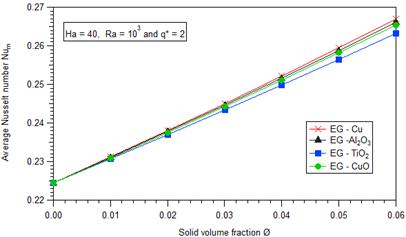Figure 15. Variation of the average Nusselt number Num according to the solid volume fraction Ø at different nanofluids for Ra = 103 , Ha = 40 and q* = 2.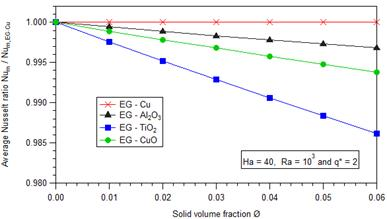Figure 16. Variation of the average Nusselt ratio Num / Num,Ethylene Glycol - cu according to the solid volume fraction Ø at different nanofluids for Ra = 103, Ha = 40 and q* = 2.

Figure 15 and 16 show, respectively, the variation of the average Nusselt number Num and the average Nusselt number ratio (Num / Num,Ethylene Glycol - cu) according to the solid volume fraction (Ø = 0.01; 0.02; 0.03; 0.04; 0.05 and 0.06) at different nanoparticles (Cu, Al2O3, CuO and TiO2) for the Hartmann number Ha = 40, the Rayleigh number Ra = 103 and the dimensionless heat generation q* = 2. The choice of the value of Rayleigh number is justified by the dominance of conduction phenomenon for this value. The thermophysical properties of the nanoparticles are given in Table 1. From these figures, we show that the heat transfer depends strongly on the nano thermal conductivity, so Ethylene Glycol-Cu nanofluid enhances the heat transfer compared with Ethylene Glycol - Al2O3, Ethylene Glycol - TiO2 and Ethylene Glycol - CuO.

5. Conclusion

Natural convection of an electrically conducting fluid in a square enclosure under an externally imposed uniform horizontal magnetic field and uniform heat generation or absorption is investigated numerically. The two opposing side walls are differentially heated with a different temperatures, whilst the floor and ceiling are thermally insulated. The coupled mass, momentum and energy equations associated are solved the commercial simulation software COMSOL Multiphysics.

The main results obtained of the numerical analysis are as follows :

For the Hartmann number (Ha = 40) and the low Rayleigh number (Ra = 103 where the conduction regime is dominant ), there is a strong clockwise circulation in the cavity for a heat sink condition (q* = - 12). But, the strength is reduced gradually when the dimensionless heat increases until high heat generation condition (q* = 12). Therefore, a higher temperature of nanofluid in the cavity which reduces the rate of heat transfer, Nusselt number and the temperature gradient near the hot wall

For the Hartmann number (Ha = 40) and the higher Rayleigh number (Ra = 107 where the convection regime is dominant ), the cavity temperature is lower than the temperature in the cavity without heat sink (in heat sink condition).

For the Hartmann number (Ha = 40) and constant solid volume fraction (Ø = 0.05) : for small Rayleigh number, the effect of heat generation (q* > 0) overcomes the effect of natural convection lead to small heat transfer from hot to cold wall or can causes reverse heat transfer from nanofluid to both vertical wall which has a negative Nusselt number. In other word, the effect of both heat generation or absorption is neglected when Rayleigh number increases.

Compared with other nanparticles, Ethylene Glycol-Cu nanofluid enhances the heat transfer which depends strongly on the nano thermal conductivity for constant Hartmann number (Ha = 40) and constant Rayleigh number (Ra = 103).

Nomenclature

B0 : magnetic field strength

Cp : specific heat

g : gravitational acceleration

h : convection heat transfer coefficient

Ha : Hartmann number

k : thermal conductivity

L : enclosure length

Nuy : local Nusselt number on the left hot wall

Num : average Nusselt number

p : fluid pressure

p* : dimensionless pressure

Pr : Prandtl number

q : heat generation/absorption

q* : dimensionless heat generation/absorption

Ra : Rayleigh number

T : temperature, K

T* : dimensionless temperature

u, v : velocity components in x, y directions

u*, v* : dimensionless velocity components

x,y : cartesian coordinates

x*, y* : dimensionless coordinates

Greek symbols

r : thermal diffusivity

b : thermal expansion coefficient

f : solid volume fraction

m : dynamic viscosity

n : kinematic viscosity

r : density

s : electrical conductivity

Y : stream function

Subscripts

c : cold wall

f : fluid

h : hot wall

nf : nanofluid

p : nanoparticle

References

1. N, Rudraiah, R.M Barron, Venkatachalappa, M., Subbaraya, C.K, "Effect of a magnetic field on free convection in a rectangular cavity", Int. J. Eng. Sci, 33, 1075-1084., 1995.
2. Piazza I. D. and Ciofalo M., "MHD free convection in a liquid-metal filled cubic enclosure. I. Differential heating",Int. J. Heat Mass Transfer,45, 1477 (2002).
3. Kandaswamy P, Sundari SM, Nithyadevi N., "Magnetoconvection in an enclosure with partially active vertical walls", Int J Heat Mass Transfer, 51, 1946–54, 2008.
4. M. Ghassemi, M. Pirmohammadi, and G. A. Sheikhzadeh, "The Effect of Magnetic Field on Buoyancy-Driven Convection in a Differentially Heated Square Cavity with Two Insulated Baffles Attached" ,Proc. of Heat Transfer Conf., ASME, Florida, pp. 141–147, 2008.
5. M. Pirmohammadi and M. Ghassemi, Effect of Magnetic Field on Convection Heat Transfer Inside a Tilted Square Enclosure,Int. Comm. in Heat and Mass Transfer, vol. 36, pp. 776–780, 2009.
6. M. Sathiyamoorthy and A. Chamkha, Effect of Magnetic Field on Natural Convection Flow in a Liquid Gallium Filled Square Cavity for Linearly Heated Side Wall,Int. J.of Therm. Sci., vol. 49, pp. 1856-1865, 2010.
7. S. Sivasankaran, C.J. Ho, Effect of temperature dependent properties on MHD convection of water near its density maximum in a square cavity, International Journal of Thermal Sciences, 47, 1184-1194, 2008.
8. Sarris, I. E., et al., MHD Natural Convection in a Laterally and Volumetrically Heated Square Cavity, International Journal of Heat and Mass Transfer, 48, 16, pp. 3443-3453, 2005.
9. Bhuvaneswari, M., Sivasankaran S., Kim, Y. J., Magneto-Convection in an Square Enclosure with Sinusoidal Temperature Distributions on Both Side Walls, Numerical Heat Transfer A, 59, 3, pp. 167-184, 2011.
10. L. Kolsi, A. Abidi, M.N. Borjini, N. Daous, H. Ben Aissia, "Effect of an External Magnetic Field on the 3-D Unsteady Natural Convection in aCubical Enclosure, Numerical Heat Transfer, Part A: Applications: An International Journal of Computation and Methodology, 51, 1003-1021, 2007.
11. H. F. Oztop and E. Abu-Nada, Numerical Study of Natural Convection in Partially Heated Rectangular Enclosures Filled with Nanofluids,Inter. J. Heat and Fluid Flow, 29, no. 5, 1326–1336, 2008.
12. E.B. Ogut, Heat transfer of water-based nanofluids with natural convection in a inclined square enclosure, Journal of Thermal Science and Technology, 30, (1) 23–33, 2010.
13. A. G. A. Nnanna, Experimental Model of Temperature-Driven Nanofluid,J. Heat Transfer, 129, no. 6, 697–704, 2007.
14. N. Putra, W. Roetzel, and S. K. Das, Natural Convection of Nano-Fluids,Heat Mass Transfer, 39, no. 8–9, 775–784, 2003.
15. D. Z. Jeng, C. S. Yang, and C. Gau, Experimental and Numerical Study of Transient Natural Convection due to Mass Transfer in Inclined Enclosures,Int. J. Heat Mass Transfer, 25, nos. 1–2, 181–192, 2008.
16. D.S. Wen, Y.L. Ding, Experimental investigation into convective heat transfer of nanofluids at entrance area under laminar flow region, Int. J. Heat Mass Transfer, 47 , 5181–5188, 2004.
17. C. J. Ho, M. W. Chen, and Z. W. Li, Numerical Simulation of Natural Convection of Nanofluid in a Square Enclosure: Effects Due to Uncertainties of Viscosity and Thermal Conductivity,Inter. J. Heat and Mass Transfer, 51, nos. 17–18, 4506–4516, 2008.
18. K. S. Hwang, J. H. Lee, and S. P. Jang, Buoyancy-Driven Heat Transfer of Water-Based Al2O3 Nanofluids in a Rectangular Cavity,Int. J. Heat Mass Transfer, 50, nos. 19–20, 4003–4010, 2007.
19. A. K. Santra, S. Sen and N. Chakraborty, "Study of Heat Transfer Augmentation in a Differentially Heated Square Cavity Using Copper-Water Nanofluid," International Journal of Thermal Sciences, 47, No. 9, 1113-1122, 2008.
20. B. Ghasemi and S. M. Aminossadati,Natural convection heat transfer in an inclined enclosure filled with a water-CuO nanofluid,Numerical Heat Transfer, Part A: Applications: An International Journal of Computation and Methodology, 55, 807-823, 2009.
21. W. Yu, H. Xie, L. Chen, Y. Li, Investigation of thermal conductivity and viscosity of ethylene glycol based ZnO nanofluid, Thermochimica Acta 491,92-96, 2009.
22. Yung-Sheng Lin , Pai-Yi Hsiao , Ching-Chang Chieng, Thermophysical characteristics of ethylene glycol-based copper nanofluids using nonequilibrium and equilibrium methods, International Journal of Thermal Sciences, 62, 56-60, 2012.
23. Eiyad Abu-Nada, Ali J. Chamkha, Effect of nanofluid variable properties on natural convection in enclosuresfilled with a CuO-EG-Water nanofluid, International Journal of Thermal Sciences, 49, 2339-2352, 2010.
24. Chan Soo Kim , Kikuo Okuyama & Juan Fernndez de la Mora Performance Evaluation of an Improved Particle Size Magnifier (PSM) for Single Nanoparticle Detection, Aerosol Science and Technology, 37, 791-803, 2003.
25. S.M. Aminossadati, B. Ghasemi, Enhanced natural convection in an isosceles triangular enclosure filled with a nanofluid, Computers and Mathematics with Applications, 61, 1739–1753, 2011.
26. Salman B.H , Mohammed H.A. , Kherbeet A. Sh, "Heat transfer enhancement of nanofluidsflow in microtube with constant heatflux", International Communications in Heat and Mass Transfer 39, 11951204, 2012.
27. Khanafer, K. M., Chamkha, A. J., Hydromagnetic Natural Convection from an Inclined Porous Square Enclosure with Heat Generation, Numerical Heat transfer A, 33, 8, pp. 891-910,
28. Hossain, M. A, Hafiz, M. Z., Rees, D. A. S., Buoyancy and Thermocapillary Driven Convection Flow of an Electrically Conducting Fluid in an Enclosure with Heat Generation, International Journal of Thermal Sciences, 44, 7, pp. 676-684, 2005.
29. Y. Xuan, W. Roetzel, Conceptions for heat transfer correlation of nanofluids,International Journal of Heat and Mass Transfer, 43 (19), 3701-3707, 2000.
30. H.C. Brinkman, The viscosity of concentrated suspensions and solution, TheJournal of Chemical Physics, 20, 571-581, 1952.
31. J.C. Maxwell, A Treatise on Electricity and Magnetism, vol. II, Oxford University Press, Cambridge, UK, p. 54, 1873.
32. Chan Soo Kim, Kikuo Okuyama, and Juan Fernandez de la Mora,Performance Evaluation of an Improved Particle Size Magnifier (PSM) for Single Nanoparticle Detection,Aerosol Science and Technology, 37, 791–803, 2003.
33. M.B. Ben Hamida and K. Charrada, Natural convection heat transfer in an enclosure filled with an Ethylene-Glycol-copper nanofluid under magnetic fields, Numerical heat transfer, part A: Applications: An international journal of computation and methodology, 67: 902–920, 2014.
34. Elif Buyuk Ogut,Natural convection of water-based nanofluids in an inclined enclosure with a heat source, International Journal of Thermal Sciences, 48, 2063–2073, 2009.
35. S. J. M. Linthorst, W. M. M. Schinkel and C. J. Hoogendoorn, J. Heat Transfer103, 535, 1981.

 Contents 1. 2. 2.1. 2.2. 2.3. 3. 4. 4.1. 4.2. 4.3. 4.4. 4.5. 5.
Article ToolsAbstractPDF(1220K)